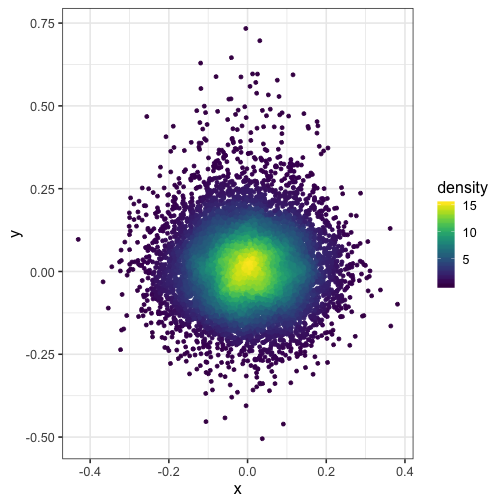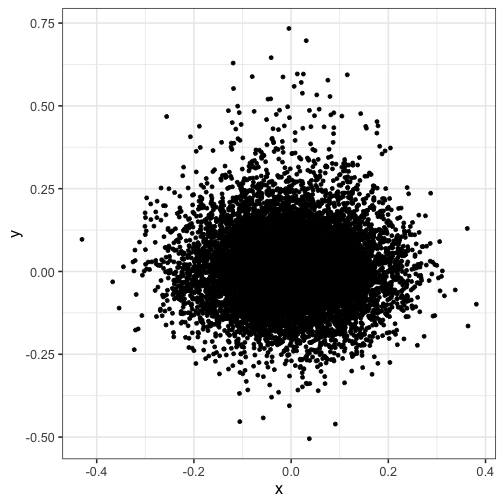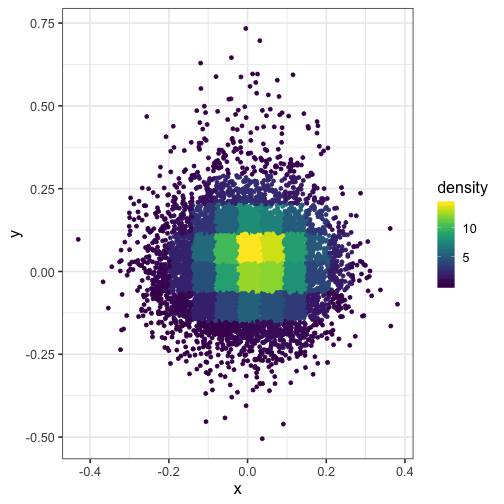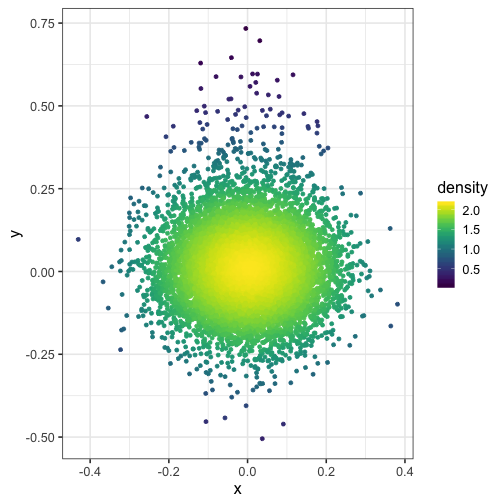# Color points by density with ggplot2

2017-01-17Here, we use the 2D kernel density estimation function from the MASS R package to to color points by density in a plot created with ggplot2. This helps us to see where most of the data points lie in a busy plot with many overplotted points.

Load libraries, define a convenience function to call MASS::kde2d, and generate some data:

library(MASS)
library(ggplot2)
library(viridis)
theme_set(theme_bw(base_size = 16))

# Get density of points in 2 dimensions.
# @param x A numeric vector.
# @param y A numeric vector.
# @param n Create a square n by n grid to compute density.
# @return The density within each square.
get_density <- function(x, y, ...) {
dens <- MASS::kde2d(x, y, ...)
ix <- findInterval(x, dens$x) iy <- findInterval(y, dens$y)
ii <- cbind(ix, iy)
return(dens$z[ii]) } set.seed(1) dat <- data.frame( x = c( rnorm(1e4, mean = 0, sd = 0.1), rnorm(1e3, mean = 0, sd = 0.1) ), y = c( rnorm(1e4, mean = 0, sd = 0.1), rnorm(1e3, mean = 0.1, sd = 0.2) ) )  Notice how the points are overplotted, so you can’t see the peak density: ggplot(dat) + geom_point(aes(x, y))Here, we split the plot into a 100 by 100 grid of squares and then color the points by the estimated density in each square. I recommend viridis for the color scheme. dat$density <- get_density(dat$x, dat$y, n = 100)
ggplot(dat) + geom_point(aes(x, y, color = density)) + scale_color_viridis()Here’s what happens when you set n = 15 (the squares in the grid are too big):

dat$density <- get_density(dat$x, dat$y, n = 15) ggplot(dat) + geom_point(aes(x, y, color = density)) + scale_color_viridis()And what if you modify the bandwidth of the normal kernel with h = c(1, 1)? dat$density <- get_density(dat$x, dat$y, h = c(1, 1), n = 100)
ggplot(dat) + geom_point(aes(x, y, color = density)) + scale_color_viridis()Check out the MASS package for more cool functions!